# WBJEE 2017 Chemistry Paper with Solutions

West Bengal Joint Entrance Examination Board conducts the WBJEE exam for admission to engineering and pharmacy courses in different colleges in the state of West Bengal. WBJEE 2017 Chemistry paper solutions are given on this page. BYJU’S provides accurate solutions that are prepared by subject experts. These solutions will help students to get an idea about the difficulty level and question pattern of the WBJEE exam. Students can download the PDF format for free to practise the paper offline.

### WBJEE 2017 - Chemistry

Question 1: For same mass of two different ideal gases of molecular weights M1 and M2, plots of log V vs log P at a given constant temperature are shown. Identify the correct option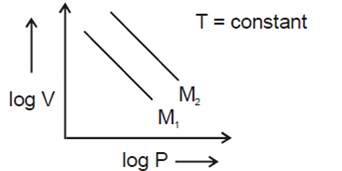1. a. M1 > M2
2. b. M1 = M2
3. c. M1 < M2
4. d. Can be predicted only if temperature is known

Solution:

Ideal gas equation PV = nRT ….(1)

Mole(x) = wt/Mw = W/M

So we can write from eq. (1) PV = (W/M) RT …..(2)

Since here w, R and T -> constant

So let w RT = k (new constant)

Now from equation (2) we get

PV = K/M ….(3)

Taking log of both side log (PV) = log (K/M)

⇒ log P + log V = log (K/M)

⇒ log V = –log P + log (K/M) …..(4) [y = mx + c]

Here + log (K/M) -> intercept

Now we can write the intercept for ideal gas (1) and (2) by the help of equation (1)

Ideal gas having M1 molar weight -> intercept = log (K/M1)

Ideal gas having M2 mw -> intercept = + log (K/M2)

From the graph we see, log (K/M2)>log(K/M1)

⇒ K/M2>K/M1

⇒ M1 > M2

Hence correct option is (a).

Question 2: Which of the following has the dimension of ML0T–2 ?

1. a. Coefficient of viscosity
2. b. Surface tension
3. c. Vapour pressure
4. d. Kinetic energy

Solution:

Surface tension = Work done per unit area

= dw/dA = F.dx/dA…..(1) {since dw = fdx}

dx -> distance /length -> m

dA -> Area -> m2

Since F = Ma = kg m/sec2….(2)

Eq. (2) -> Eq. (1)

Surface tension = (kg m/sec2) × m/m2 = kg sec–2

Therefore the dimension of the surface tension is

[M1L0T–2]

Hence correct option (b).

Question 3: If the given four electronic configurations

(i) n = 4, l = 1 (ii) n = 4, l = 0 (iii) n = 3, l = 2 (iv) n = 3, l = 1

are arranged in order of increasing energy, then the order will be

1. a. (iv) < (ii) < (iii) < (i)
2. b. (ii) < (iv) < (i) < (iii)
3. c. (i) < (iii) < (ii) < (iv)
4. d. (iii) < (i) < (iv) < (ii)

Solution:

According n + l rule, n + l value increases, energy of orbital also increases

Hence (1) n + l = 4 + 1 = (5) ⇒ 4p orbital

(2) n + l = 4 + 0 = (4) ⇒ 4s orbital

(3) n + l = 3 + 2 = (5) ⇒ 3d orbital

(4) n + l = 3 + 1 = (4) ⇒ 3p orbital

If for any two cases n + l values are equal, then whose orbital which have lower n value, their orbital energy also lower.

Hence overall order of energy = 1 > 3 > 2 > 4

So correct option is (a).

Question 4: Which of the following sets of quantum numbers represents the 19th electron of Cr (Z = 24)?

1. a. (4,1,-1,+1/2)
2. b. (4,0,0,+1/2)
3. c. (3,2,0,-1/2)
4. d. (3,2,-2,+1/2)

Solution:

Electronic configuration of Cr (Atomic number 24)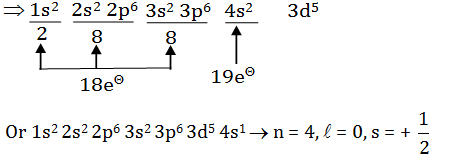Here 19e- enter in 4s orbital because 4s orbital have lower energy compared to 3d orbital

Hence correct option is (b).

Question 5: 0.126 g of an acid is needed to completely neutralize 20 ml 0.1 (N) NaOH solution. The equivalent weight of the acid is

1. a. 53
2. b. 40
3. c. 45
4. d. 63

Solution:

Number of eq. of acid = No. of eq. of base

= N × V/1000 = 0.1 × 20/1000 = 2 × 10–3

N = No. of eq. of solute /V(litre)

⇒2×10–3 equivalents have mass = 0.126 g

Hence, mass of 1 equivalent = 0.126/2 ×10-3 = 63 g

So correct option is (d).

Question 6: In a flask, the weight ratio of CH4 (g) and SO2(g) at 298 K and 1 bar is 1 : 2. The ratio of the number of molecules of SO2(g) and CH4(g) is

1. a. 1 : 4
2. b. 4 : 1
3. c. 1 : 2
4. d. 2 : 1

Solution:

Wt. ratio WCH4 : WSO2 = 1:2

Moles (n) = wt/Mw

Mw of SO2 = 64 g mol-1

Mw of CH4 = 16 g mol-1

nSO2/nCH4 = (WSO2 ×MCH4 )/ (MSO2 ×WCH4 )

So mole ratio of SO2: CH4 = 1 : 2

Hence correct option is c.

Question 7: C6H5F18 is a F18 radio-isotope labeled organic compound. F18 decays by positron emission. The product resulting on decay is :

1. a. C6H5O18
2. b. C6H5Ar19
3. c. B12C5H5F
4. d. C6H5O16

Solution:

Positron emission by 9F18 follows as

9F18 -> 8O18 + +1e0

When an element emit a positron [Beta particle (β+)] decreases the no. of proton by one. and increases the neutron by one. While mass number A remains the same.

So resulting product is as ⇒ C6 H6 O18

Hence correct option is (a).

Question 8: Dissolving NaCN in de-ionized water will result in a solution having

1. a. pH < 7
2. b. pH = 7
3. c. pOH = 7
4. d. pH > 7

Solution:

On dissolving NaCN

NaCN + H2O -> NaOH (strong base) +HCN ->Weak acid

Hence pH > 7

So correct option is (d).

Question 9: Among Me3N, C5H5N and MeCN (Me = methyl group) the electronegativity of N is in the order

1. a. MeCN > C5H5N > Me3N
2. b. C5H5N > Me3N > MeCN
3. c. Me3N > MeCN > C5H5N
4. d. Electronegativity same in all

Solution:

As the % S increases electronegativity increases.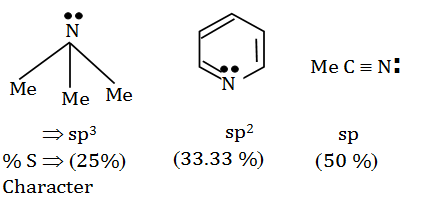Hence order of E.N. MeCN > C5H5N > Me3N

So correct option is (a).

Question 10: The shape of XeF5 will be

1. a. Square pyramid
2. b. Trigonal bipyramidal
3. c. Planar
4. d. Pentagonal bipyramid

Solution:

Shape of XeF5-:

No. of electron pair = (8+5+1)/2 = 7 -> sp3d3 hybridisation

⇒ np +l p = 7

l p = 7–5 = 2

l p = 2

Shape of molecule which have sp3d3 hybridisation and 2lp

l p present at axial to minimize the repulsion

⇒ Hence shape is planar & geometry pentagonal bipyramidal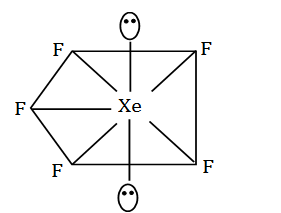Geometry -> Pentagonal bipyramidal

Shape -> planar

Question 11: The ground state magnetic property of B2 and C2 molecules will be

1. a. B2 paramagnetic and C2 diamagnetic
2. b. B2 diamagnetic and C2 paramagnetic
3. c. Both are diamagnetic
4. d. Both are paramagnetic

Solution:

MOT diagram of C2 molecule ⇒ 6C -> 1s22s22p2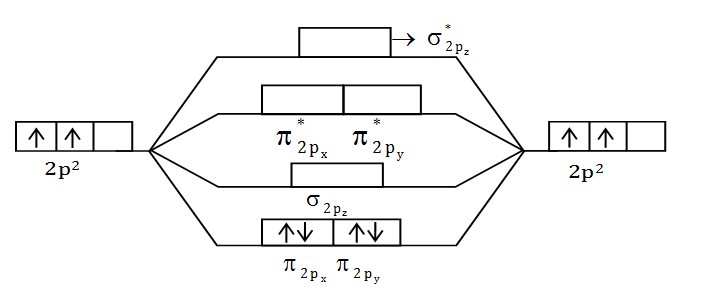Unpaired electron = 0

MM = 0 BM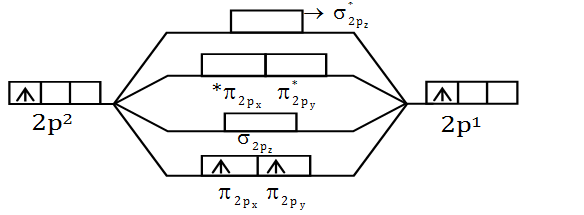No. of unpaired electron = 2

MM = √(n(n+2) = √(2(2+2) = √8

M.M = 2.83 BM

Hence C2 diamagnetic & B2 paramagnetic

So correct option is (a).

Question 12: The number of unpaired electrons in [NiCl4]2–, Ni(CO)4 and [Cu(NH3)4]2+ respectively are

1. a. 2, 2, 1
2. b. 2, 0, 1
3. c. 0, 2, 1
4. d. 2, 2, 0

Solution:

(1)[NiCl4]2– -> Ni+2 -> 3d8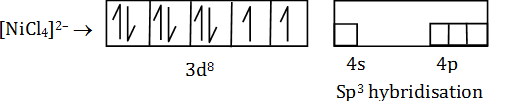Since Cl- is a weak ligand, so there is no pairing of electrons

No. of unpaired electron = 2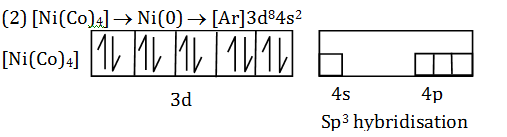Since Co is a strong ligand, so there is pairing of electrons occurs

No. of unpaired electrons = 0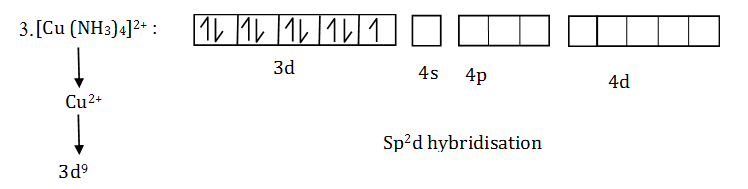No. of unpaired electrons = 1

Hence correct option is (b).

Question 13: Which of the following atoms should have the highest 1st electron affinity?

1. a. F
2. b. O
3. c. N
4. d. C

Solution:

Electro affinity ⇒ E.A. of an atom is defined as the amount of energy released when an electron is attached to a neutral atom or molecule in the gaseous state to form a negative ion.

x(g) + e- -> xg- + energy

E.A. of “F” is 2nd highest amongst periodic table after “Cl”

Question 14: PbCl2 is insoluble in cold water. Addition of HCl increases its solubility due to

1. a. Formation of soluble complex anions like [PbCl3]–
2. b. Oxidation of Pb(II) to Pb (IV)
3. c. Formation of [Pb(H2O)6]2+
4. d. Formation of polymeric lead complexes

Solution:

Solubility of PbCl2 increases in cold water on the addition of HCl due to the formation of soluble complex like [PtCl3]- (aq.).

PbCl2 react with HCl as follows

PbCl2(s) + Cl- -> [PbCl3]- (aq)

PbCl2(s) + 2Cl- -> [PbCl4]2– (aq)

Thus the addition of excess amount of Cl- ions change the PbCl2 as soluble complex of [PbCl4]2-.

Hence solubility increases .

Question 15: Of the following compounds, which one is the strongest Bronsted acid in aqueous solution?

1. a. HClO3
2. b. HClO2
3. c. HOCl
4. d. HOBr

Solution:

As the oxidation state of Central atom increases Electron negativity increases and acidity increases.

I effect increases, acidity increases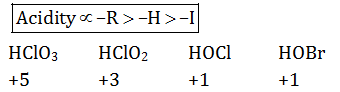Hence correct option is (a).

Question 16: The correct basicity order of the following lanthanide ions is

1. a. La3+ > Lu3+ > Ce3+ > Eu3+
2. b. Ce3+ > Lu3+ > La3+ > Eu3+
3. c. Lu3+ > Ce3+ >Eu3+ > La3+
4. d. La3+ > Ce3+ > Eu3+ > Lu3+

Solution:

Due to the lanthanoid contraction, increase in atomic number the basicity decrease from La3+ to Lu3+.

So correct order of basicity follow as La3+ > Ce3+ > Eu3+ > Lu3+.

Hence correct option is (d).

Question 17: When BaCl2 is added to an aqueous salt solution, a white precipitate is obtained. The anion among CO32– , SO32– and SO42– that was present in the solution can be :

1. a. CO32– but not any of the other two
2. b. SO32– but not any of the other two
3. c. SO42– but not any of the other two
4. d. Any of them

Solution:

Reaction of BaCl2 with CO32– , SO32– & SO42– follow as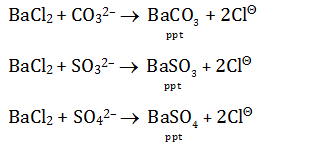Hence correct option is (d).

Question 18: In the IUPAC system, PhCH2CH2CO2H is named as

1. a. 3-phenylpropanoic acid
2. b. benzylacetic acid
3. c. carboxyethylbenzene
4. d. 2-phenylpropanoic acid

Solution: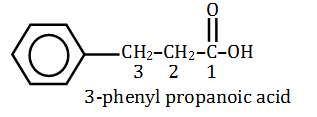Hence correct option (a).

Question 19: The isomerisation of 1-butyne to 2-butyne can be achieved by treatment with

1. a. hydrochloric acid
2. b. ammonical silver nitrate
3. c. ammonical cuprous chloride
4. d. ethanolic potassium hydroxide

Solution:

Isomerisation of 1-butyne to 2-butyne can be achived by treatment with ethanolic KOH.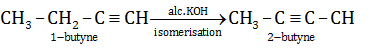Question 20: The correct order of acid strengths of benzoic acid (X), peroxybenzoic acid (Y) and p-nitrobenzoic acid (Z) is

1. a. Y > Z > X
2. b. Z > Y > X
3. c. Z > X > Y
4. d. Y > X > Z

Solution: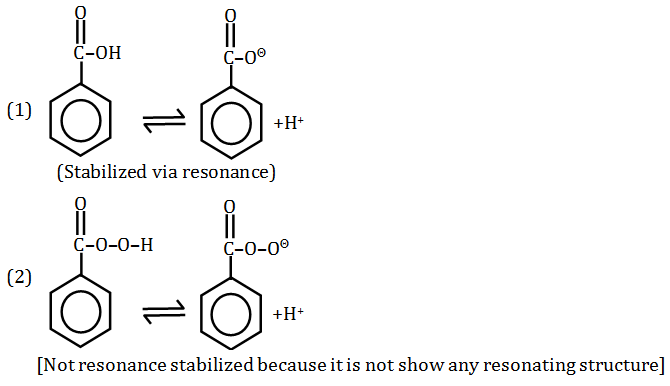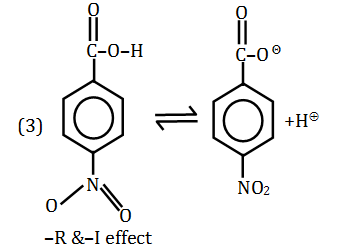[Most acidic due to –R & –I effect of –NO2 group]

Acidity ∝ -R>-H>-I

Hence correct order of acidity is 3 > 1 > 2

So correct option is (c)

Question 21: The yield of acetanilide in the reaction (100% conversion) of 2 moles of aniline with 1 mole of acetic anhydride is

1. a. 270 g
2. b. 135 g
3. c. 67.5 g
4. d. 177 g

Solution:

The reaction of 2 moles with 1 mole of acetic anhydride follows as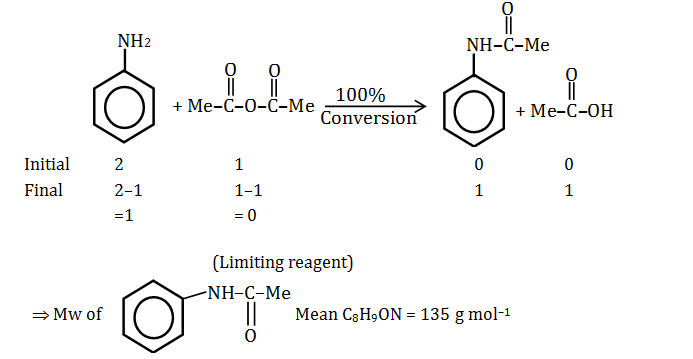Wt. of acetanilide = moles × Mw = 1 × 135

= 135 g

Hence correct option is (b).

Question 22: The structure of the product P of the following reaction is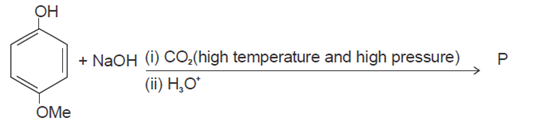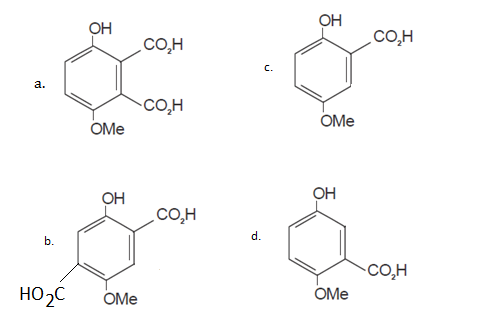Solution:

Reaction mechanism follows as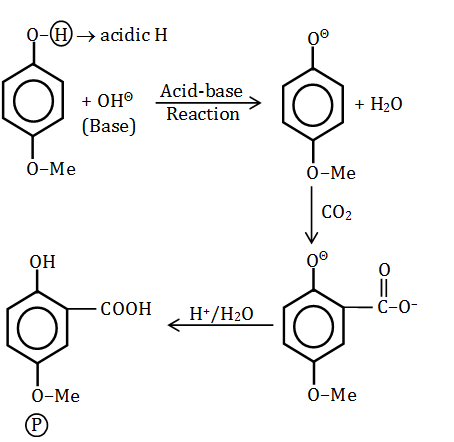Question 23: ADP and ATP differ in the number of

1. a. phosphate units
2. b. ribose units
3. c. adenine base
4. d. nitrogen atom

Solution:

ADP ⇒ Adenosine diphosphate ⇒ 2 phosphate group

ADP ⇒ Adenosine Triphosphate ⇒ 3 phosphate group

⇒ So difference only one phosphate group

Hence correct option is (a).

Question 24: The compound that would produce a nauseating smell/odour with a hot mixture of chloroform and ethanolic potassium hydroxide is

1. a. PhCONH2
2. b. PhNHCH3
3. c. PhNH2
4. d. PhOH

Solution:

Carbyl – amine reaction

When CHCl3 + KOH (alcoholic), React with 10 amine

Or aromatic amine: Isocyanide product will form, reaction follow as –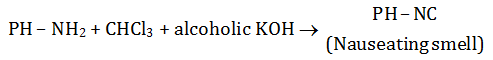Hence correct option is (c).

Question 25: For the reaction below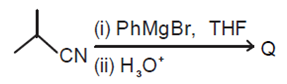the structure of the product Q is: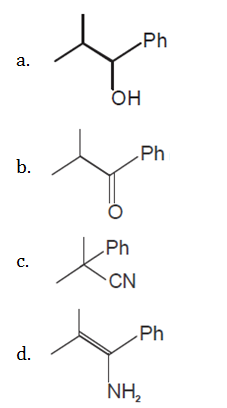Solution:

Reaction mechanism follow as :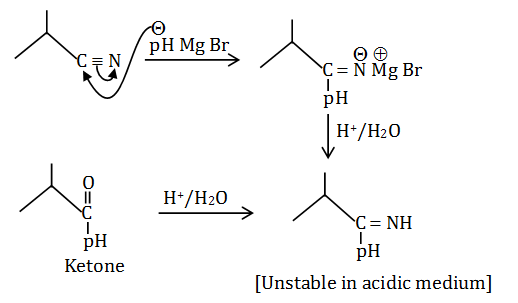Question 26: You are supplied with 500 ml each of 2N HCl and 5N HCl. What is the maximum volume of 3M HCl that you can prepare using only these two solutions?

1. a. 250 ml
2. b. 500 ml
3. c. 750 ml
4. d. 1000 ml

Solution:

We have to prepare maximum volume solution of 3N. {3 M is same as 3 N for HCl due to valency factor = 1}

Hence we take 500 ml of 2N solution & x ml of 5N solution

N1V1 + N2V2 = N3V3

⇒500 × 2 + x × 5 = 3 (x + 500)

1000+5x = 3x+1500

x = 250 ml

Hence final volume of the solution = x + 500 = 250 + 500 = 750 ml

Question 27: Which one of the following corresponds to a photon of highest energy?

1. a. λ = 300 nm
2. b. ν = 3 × 108 s–1
3. c. ν = 30 cm–1
4. d. ε = 6.626 × 10–27 J

Solution:

Energy of photon (E) = h ν = hc/λ

(1) E = hc/λ = (6.626 ×10-34J S×3×108m sec-1)/300×10-9m

E = 6.626 ×10-19J

(2) E = h ν =6.626 ×10–34 J. sec × 3 × 108 sec–1

E = 1.9878 ×10-25J

(3) E = chν = 6.626 × 10–34 J sec×30×102 m–1×3 ×108 m sec–1

= 5.9634 ×10–22 J

(4) E = 6.626 × 10–27 J

Among these, maximum energy = 6.626×10–19 J

Hence correct option is (A)

*v is representing "nu" here.

Question 28: Assuming the compounds to be completely dissociated in aqueous solution, identify the pair of the solutions that can be expected to be isotonic at the same temperature :

1. a. 0.01 M Urea and 0.01 M NaCl
2. b. 0.02 M NaCl and 0.01 M Na2SO4
3. c. 0.03 M NaCl and 0.02 M MgCl2
4. d. 0.01 M Sucrose and 0.02 M glucose

Solution:

For isotonic at some temperature π1 = π2

i1c1RT = l2c2RT …..(1)

Option (c) 0.03 M NaCl & 0.02 M MgCl2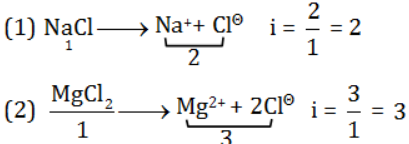Now from equation (1)

2 × 0.03 = 0.06

3× 0.02 = 0.06

So i1c1 = i2c2.

Hence it is isotonic.

Question 29: How many faradays are required to reduce 1 mol of Cr2O72– to Cr3+ in acid medium?

1. a. 2
2. b. 3
3. c. 5
4. d. 6

Solution: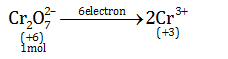Hence reduction of 1 mol required 6 electron

So no. of the Faraday’s = 6F

Question 30: Equilibrium constants for the following reactions at 1200 K are given :

2H2O(g) ⇌ 2H2(g) + O2(g) ; K1 = 6.4 × 10–8

2CO2(g) ⇌ 2CO(g) + O2(g); K2 = 1.6 × 10–6

The equilibrium constant for the reaction

H2(g) + CO2(g) ⇌ CO(g) + H2O(g) at 1200 K will be

1. a. 0.05
2. b. 20
3. c. 0.2
4. d. 5.0

Solution: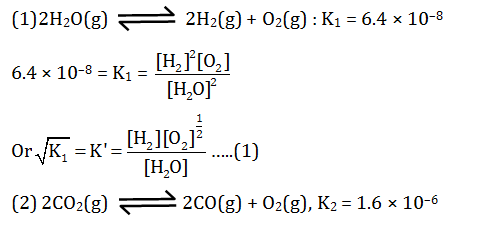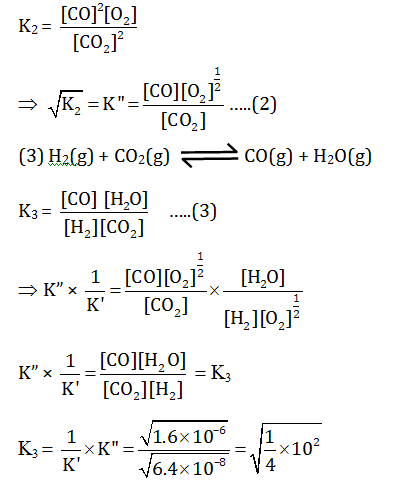K3 = 5

Hence correct option is (d).

Question 31: In a close-packed body-centred cubic lattice of potassium, the correct relation between the atomic radius (r) of potassium and the edge-length (a) of the cube is

1. a. r = a/√2
2. b. r = a/√3
3. c. r = √3/2a
4. d. r = √3/4a

Solution:

Here, we have √3a = 4r

r = √3a/4

(Where a = edge length, r = radius of lattice sphere)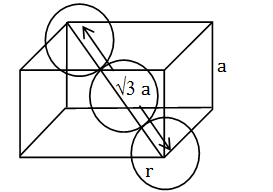Question 32: Which of the following solutions will turn violet when a drop of lime juice is added to it?

1. a. A solution of Nal
2. b. A solution mixture of KI and NalO3
3. c. A solution mixture of Nal and KI
4. d. A solution mixture of KIO3 and NalO3

Solution:

Lime Juice is acidic in nature as it contains “citric acid”. The citric acid present in lime juice will liberate I2 from the iodide which will give purple or violet colour.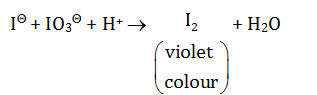Question 33: The reaction sequence given below gives product R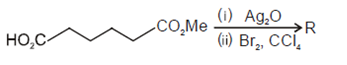The structure of the product R is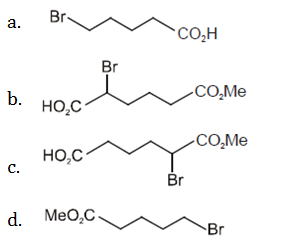Solution:

Reaction mechanism of the reaction follows as

This reaction is an example of “Borodine” Huns Diecker reaction.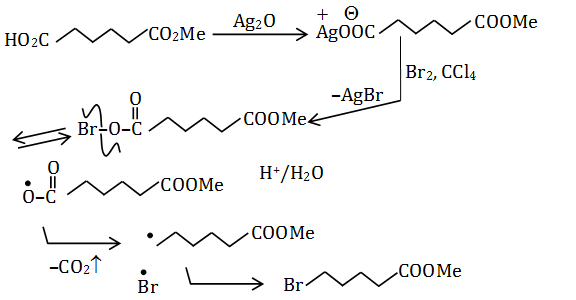Question 34: Reduction of the lactol S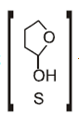with sodium borohydride gives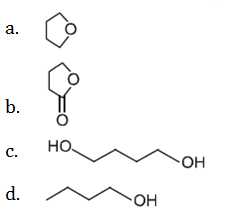Solution:

The reduction reaction of lactol (s) with sodium borohydride (NaBH4)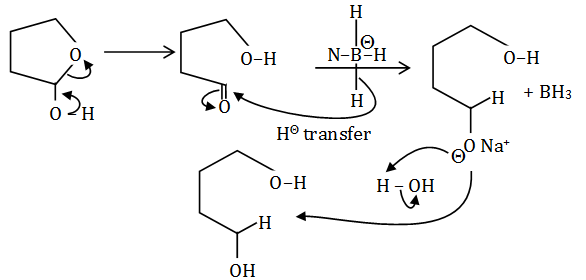Hence correct option is (c).

Question 35: What will be the normality of the salt solution obtained by neutralizing x ml y (N) HCl with y ml x (N) NaOH, and finally adding (x + y) ml distilled water?

1. a. 2(x+y)N/xy
2. b. xyN/2(x+y)
3. c. 2xyN/(x+y)
4. d. (x+y)N/xy

Solution:

Normality = wt × 1000/eq.wt ×v(ml) = No. of M.eq/volume of solution (ml)

Reaction between NaOH & HCl follow as

It is an acid-base reaction hence final product is salt & H2O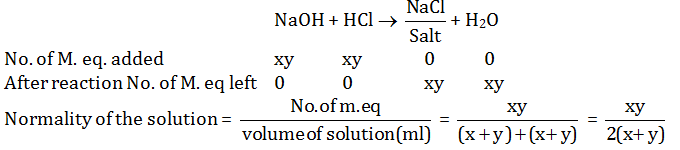Question 36: During electrolysis of molten NaCl, some water was added. What will happen?

1. a. Electrolysis will stop
2. b. Hydrogen will be evolved
3. c. Some amount of caustic soda will be formed
4. d. A fire is likely

Solution:

Electrolysis of molten NaCl

Molten NaCl (An electrolyte) means free Na+ ions & Cl- ion, so it is conducts current with the help of ions. As electric current is passed in the cell, Cl- ions are attracted to the anode (positive) & Na+ ions attracted to cathode (negative).

Anode to cathode follow as :

Cathode Na+ + e- -> Na(a)

Anode Cl- -> ½ Cl2 + e-

Overall reaction Na+ +Cl- -> Na + ½ Cl2

If we add some water then, H2 will liberate & NaOH will form in the solution and reaction follows as

Exothermic reaction

Na(s) + H2O -> NaOH + (1/2)H2 +Q (energy)

Hence correct option is (b,c,d).

Question 37: The role of fluorspar, which is added in small quantities in the electrolysis reduction of alumina dissolved in fused cryolite, is

1. a. as a catalyst
2. b. to make fused mixture conducting
3. c. to lower the melting temperature of the mixture
4. d. to decrease the rate of oxidation of carbon at anode

Solution:

The electrolysis of Al2O3 is carried out in the steel tank linked inside with graphite. The graphite lining serves as cathode. The anode is also made of graphite rods hanging in the molten mass the electrolyte consist of alumina dissolved in fused cryolite (Na3AlF6) & CaF2. Cryolite lower the melting point of alumina to 950°C and CaF2 increase the fluidity of the mass, so that the liberated aluminium metal may sink at the bottom of the cell. Therefore, it makes the fused mixture very conducting & lower the fusion temperature of the metal. When an electric current is passed through this mixture the Al is collected at the cathode in the molten state & sinks at the bottom & it is tapped off.

Hence correct option is (b,c).

Question 38:The reduction of benzenediazonium chloride to phenyl hydrazine can be accomplished by

1. a. SnCl2, HCl
2. b. Na2SO3
3. c. CH3CH2OH
4. d. H3PO2

Solution:

Reaction diazonium salt with following reagent follow as :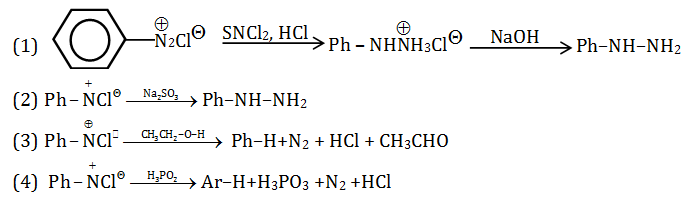Hence correct option are (a,b)

Question 39: The major product(s) obtained from the following reaction of 1 mole of hexadeuterobenzene is/are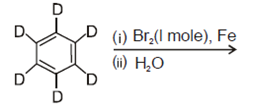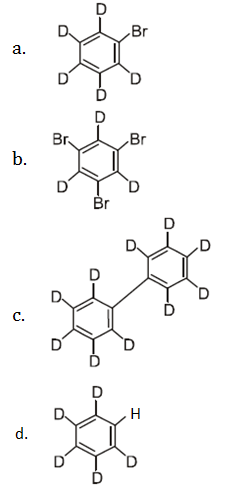Solution: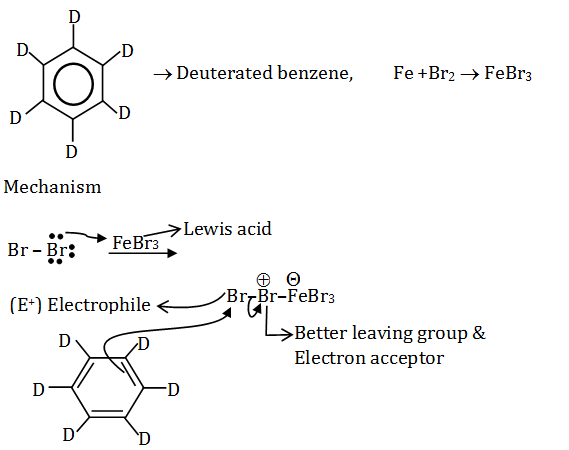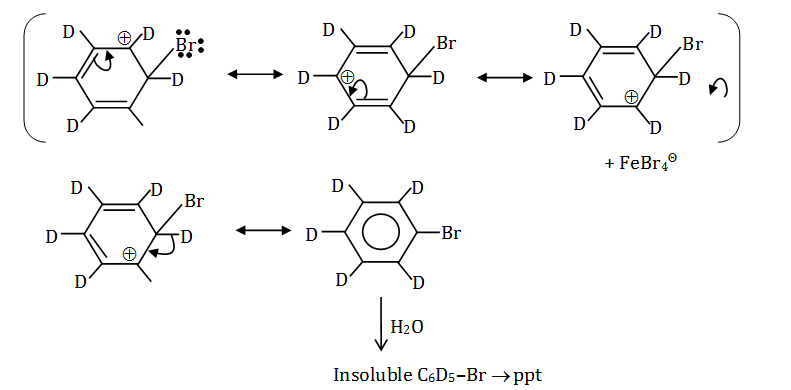Hence correct option is (a).

Question 40: Identify the correct statement(s) :

The findings from the Bohr model for H-atom are

1. a. Angular momentum of the electron is expressed as integral multiples of h/2 π
2. b. The first Bohr radius is 0.529A0
3. c. The energy of the n-th level En is proportional to 1/n2
4. d. The spacing between adjacent levels increases with increase in ‘n’

Solution:

(A) Angular momentum = nh/2π where [n = 1, 2, 3]

(B) rn = 0.529 ×n2/z Å

For H, z = 1

⇒ n = 1 (according question)

r = 0.529Å

(C) En = – 13.6 × z2/n2 eV/atom

En ∝ 1/n2

(D) From the above reaction

E1 = – 13.6 eV/atom

E2 = – 13.6 ×z2/22 = –3.4 eV/atom

E3 = – 13.6/9 = 1.51 ev atom

E2 – E1 = 10.2 ev/atom

E3 –E2 = 0.89 ev /atom

So (E2–E1) > (E3–E2) > (E4–E3) ……

As the difference between the successive energy level decreases, “n” increases.

Hence, correct option is (a,b,c).

### WBJEE 2017 Chemistry Question Paper with Solutions